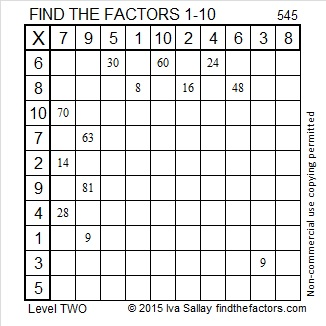# 554 and Level 4

554 is the hypotenuse of the Pythagorean triple 230-504-554. What is the greatest common factor of those three numbers?Print the puzzles or type the solution on this excel file: 12 Factors 2015-07-13

—————————————————————————————————

• 554 is a composite number.
• Prime factorization: 554 = 2 x 277
• The exponents in the prime factorization are 1 and 1. Adding one to each and multiplying we get (1 + 1)(1 + 1) = 2 x 2 = 4. Therefore 554 has exactly 4 factors.
• Factors of 554: 1, 2, 277, 554
• Factor pairs: 554 = 1 x 554 or 2 x 277
• 554 has no square factors that allow its square root to be simplified. √554 ≈ 23.5372—————————————————————————————————Advertisements

# 553 and Level 3

79 is a factor of 553. If you start at 79 and go backwards adding all the prime numbers from 79 to 43, the sum will be 553. Do you know those prime numbers forwards and backwards well enough to do that?Print the puzzles or type the solution on this excel file: 12 Factors 2015-07-13

—————————————————————————————————

• 553 is a composite number.
• Prime factorization: 553 = 7 x 79
• The exponents in the prime factorization are 1 and 1. Adding one to each and multiplying we get (1 + 1)(1 + 1) = 2 x 2 = 4. Therefore 553 has exactly 4 factors.
• Factors of 553: 1, 7, 79, 553
• Factor pairs: 553 = 1 x 553 or 7 x 79
• 553 has no square factors that allow its square root to be simplified. √553 ≈ 23.51595—————————————————————————————————

A Logical Approach to solve a FIND THE FACTORS puzzle: Find the column or row with two clues and find their common factor. Write the corresponding factors in the factor column (1st column) and factor row (top row).  Because this is a level three puzzle, you have now written a factor at the top of the factor column. Continue to work from the top of the factor column to the bottom, finding factors and filling in the factor column and the factor row one cell at a time as you go.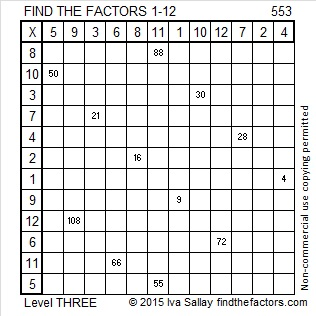# Level 2 and Simplifying √552

552 is the sum of all the prime numbers from 37 to 73.

It is also the sum of all the prime numbers from 79 to 103.

That means if you add up all the prime numbers from 37 to 103, you’ll get 1104 which is 2 x 552.

Can you list all those prime numbers?Print the puzzles or type the solution on this excel file: 12 Factors 2015-07-13

—————————————————————————————————

I’ve modified the cake method to help me find √552.  My philosophy is that there is no reason to completely break up 552 to find its square root when we’re just going to have to put it mostly back together again to give the final answer. About 83% of numbers whose square roots can be reduced are divisible by 4 and/or 9. It makes sense to check to see if 552 is divisible by either of those numbers first.

552 can be evenly divided by 4 because its last two digits are divisible by 4. That means √552 can be reduced. Let’s find 552 ÷ 4.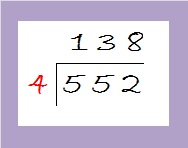The quotient is 138 which is even but not divisible by 4. Let’s check to see if it is divisible by nine: 1 + 3 + 8 = 12. It is divisible by 3, but not by 9.

Since 138 is even and divisible by 3, it is divisible by 6. It is easier to divide by 6 once than it is to divide first by 2 and then by 3. Dividing by 6 makes our division cake look like this: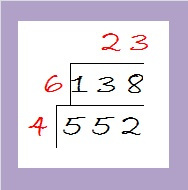Since 23 is a prime number, we are done with the division process. Taking the square root of everything on the outside of the cake we get √552 = (√4)(√6)(√23) = (√4)(√138) = 2√138.

—————————————————————————————————

• 552 is a composite number.
• Prime factorization: 552 = 2 x 2 x 2 x 3 x 23, which can be written 552 = (2^3) x 3 x 23
• The exponents in the prime factorization are 3, 1, and 1. Adding one to each and multiplying we get (3 + 1)(1 + 1)(1 + 1) = 4 x 2 x 2 = 16. Therefore 552 has exactly 16 factors.
• Factors of 552: 1, 2, 3, 4, 6, 8, 12, 23, 24, 46, 69, 92, 138, 184, 276, 552
• Factor pairs: 552 = 1 x 552, 2 x 276, 3 x 184, 4 x 138, 6 x 92, 8 x 69, 12 x 46, or 23 x 24
• Taking the factor pair with the largest square number factor, we get √552 = (√4)(√138) = 2√138 ≈ 23.49468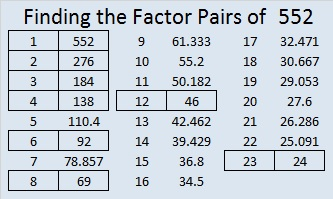—————————————————————————————————# 551 and Level 1

551 is the sum of all the prime numbers from 11 to 67. It also equals this sum of consecutive primes: 179 + 181 + 191.

551 is the hypotenuse of the Pythagorean triple 380-399-551. What is the greatest common factor of those three numbers? Hint: It’s one of the factors of 551 listed below the puzzle.Print the puzzles or type the solution on this excel file: 12 Factors 2015-07-13

—————————————————————————————————

• 551 is a composite number.
• Prime factorization: 551 = 19 x 29
• The exponents in the prime factorization are 1 and 1. Adding one to each and multiplying we get (1 + 1)(1 + 1) = 2 x 2 = 4. Therefore 551 has exactly 4 factors.
• Factors of 551: 1, 19, 29, 551
• Factor pairs: 551 = 1 x 551 or 19 x 29
• 551 has no square factors that allow its square root to be simplified. √551 ≈ 23.453389—————————————————————————————————# 550 Godparents

All of these children are more puzzle pieces in the life of Kéri Mihály (Michael Keri).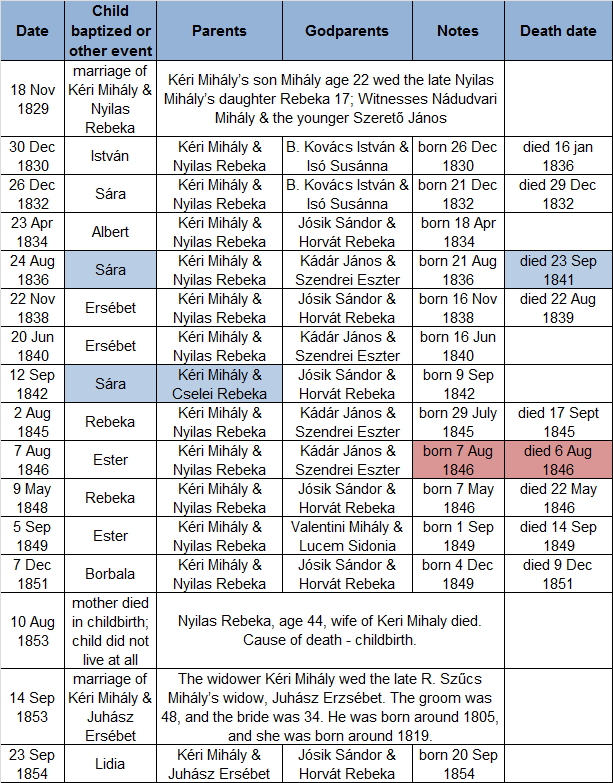I’m sharing this chart even though I have not yet found all of the children’s death dates. The highlighted entries will help me explain a thing or two.

The parents listed for the Sára christened in 1842 are Kéri Mihály and Cselei Rebeka (highlighted in blue). I believe the minister made a mistake writing Cselei instead of Nyilas. Here are my reasons:

1. I didn’t find a Kéri-Cselei marriage record or any other children for a couple with those names.
2. Kéri Mihály and Nyilas Rebeka had a child every two to three years. There would be a five year gap if 1842 Sára is not included in the family.
3. The couple had a previous child they named Sára who died in 1841, a year before 1842 Sára was born.
4. 1842 Sára’s godparents were also the godparents of five of her siblings. I looked to see if Michael Keri and Rebeka were the godparents for the Sandor Josik and Rebeka Horvat’s children. They weren’t, but Sandor Josik and Rebeka Horvat also were not the godparents for any other couple from 1841 to 1843.

Another mistake was obviously made recording dates for Ester who has some conflicting dates highlighted in red. I double checked all the information when I added it to the chart. If you were to follow the christening record and the death record, Ester was born on the 7th, christened on the 7th, died on the 6th, and buried on the 8th. Her death record also stated that she was 3 days old when she died. Obviously at least one of the dates is not correct.

Life must have been very difficult for Michael and Rebeka Keri. A little baby usually represents much hope for the future. This couple had to witness the deaths of too many of their little ones. My heart goes out to them.

————————————————————————–

550 is the product of 10 and the 10th triangular number and is, therefore, the 10th pentagonal pyramidal number.

550 is the hypotenuse of two Pythagorean triples: 330-440-550 and 154-528-550. What is the greatest common factor of each of those triples?

• 550 is a composite number.
• Prime factorization: 550 = 2 x 5 x 5 x 11, which can be written 550 = 2 x (5^2) x 11
• The exponents in the prime factorization are 1, 2, and 1. Adding one to each and multiplying we get (2 + 1)(1 + 1)(1 + 1) = 3 x 2 x 2 = 12. Therefore 550 has exactly 12 factors.
• Factors of 550: 1, 2, 5, 10, 11, 22, 25, 50, 55, 110, 275, 550
• Factor pairs: 550 = 1 x 550, 2 x 275, 5 x 110, 10 x 55, 11 x 50, or 22 x 25
• Taking the factor pair with the largest square number factor, we get √550 = (√25)(√22) = 5√22 ≈ 23.452079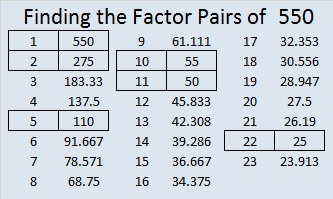# 549 and Level 6

549 is the hypotenuse of the Pythagorean triple 99-540-549. What is the greatest common factor of those three numbers?Print the puzzles or type the solution on this excel file: 10 Factors 2015-07-06

—————————————————————————————————

• 549 is a composite number.
• Prime factorization: 549 = 3 x 3 x 61, which can be written 549 = (3^2) x 61
• The exponents in the prime factorization are 2 and 1. Adding one to each and multiplying we get (2 + 1)(1 + 1) = 3 x 2  = 6. Therefore 549 has exactly 6 factors.
• Factors of 549: 1, 3, 9, 61, 183, 549
• Factor pairs: 549 = 1 x 549, 3 x 183, or 9 x 61
• Taking the factor pair with the largest square number factor, we get √549 = (√9)(√61) = 3√61 ≈ 23.430749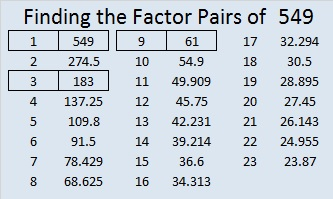—————————————————————————————————# 548 and Level 5

548 is the hypotenuse of the Pythagorean triple 352-420-548. What is the greatest common factor of those three numbers?Print the puzzles or type the solution on this excel file: 10 Factors 2015-07-06

—————————————————————————————————

• 548 is a composite number.
• Prime factorization: 548 = 2 x 2 x 137, which can be written 548 = (2^2) x 137
• The exponents in the prime factorization are 2 and 1. Adding one to each and multiplying we get (2 + 1)(1 + 1) = 3 x 2  = 6. Therefore 548 has exactly 6 factors.
• Factors of 548: 1, 2, 4, 137, 274, 548
• Factor pairs: 548 = 1 x 548, 2 x 274, or 4 x 137
• Taking the factor pair with the largest square number factor, we get √548 = (√4)(√137) = 2√137 ≈ 23.4093998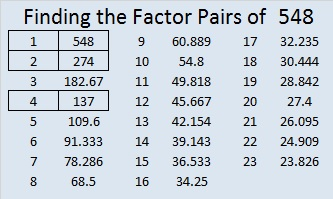—————————————————————————————————# 547 and Level 4

547 is the difference of two consecutive numbers that have been cubed.

Thus 547 = 14³ – 13³.We can use divisibility tricks that easily show that 547 is not divisible by 2, 3, 5, or 11.

• 547 is odd so it’s not divisible by 2
• 5 + 4 + 7 = 16 which is not divisible by 3, so 547 is not divisible by 3
• The last digit of 547 is not 5 or 0 so it is not divisible by 5
• 5 – 4 + 7 = 8 which is not divisible by 11, so 547 is not divisible by 11

Also because it is the difference of 14^3 and 13^3, and 13 and 14 have no common prime factors, 547 also cannot be evenly divided by 13, 14 or any of their prime factors (2 and 7). Thus 547 is not divisible by 2, 3, 5, 7, 11, or 13. Could 547 possibly be a prime number? We only have to try to divide it by 17, 19, and 23 to know for sure! Scroll down past the puzzle to find out.Print the puzzles or type the solution on this excel file: 10 Factors 2015-07-06

—————————————————————————————————

• 547 is a prime number.
• Prime factorization: 547 is prime.
• The exponent of prime number 547 is 1. Adding 1 to that exponent we get (1 + 1) = 2. Therefore 547 has exactly 2 factors.
• Factors of 547: 1, 547
• Factor pairs: 547 = 1 x 547
• 547 has no square factors that allow its square root to be simplified. √547 ≈ 23.38803How do we know that 547 is a prime number? If 547 were not a prime number, then it would be divisible by at least one prime number less than or equal to √547 ≈ 23.4. Since 547 cannot be divided evenly by 2, 3, 5, 7, 11, 13, 17, 19, or 23, we know that 547 is a prime number.

—————————————————————————————————# 546 and Level 3

546 is made from 3 consecutive numbers so it is divisible by 3.

The sum of all the prime numbers from 53 to 83 is 546. Can you list all those prime numbers?

546 is the hypotenuse of the Pythagorean triple 210-504-546. Those three numbers have eight common factors, but what is the greatest common factor?

—————————————————————————————————

Puzzles can be a great resource in teaching mathematics. I’ve made crossword puzzles to review vocabulary words before, but none of them looked as inviting to complete as the one Resourceaholic recommended in her 7 July 2015 post on end of term resources. What a difference a little clip art makes to a well-constructed crossword puzzle! The post also includes links to several other amazing mathematics-related puzzles including a polygon word search and a number sequence number search puzzle. Check it out!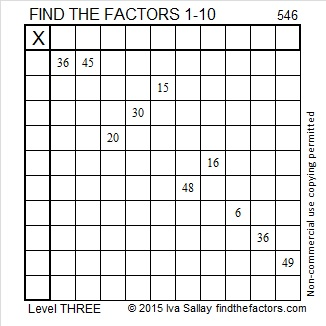Print the puzzles or type the solution on this excel file: 10 Factors 2015-07-06

—————————————————————————————————

• 546 is a composite number.
• Prime factorization: 546 = 2 x 3 x 7 x 13
• The exponents in the prime factorization are 1, 1, 1, and 1. Adding one to each and multiplying we get (1 + 1)(1 + 1)(1 + 1)(1 + 1) = 2 x 2 x 2 x 2 = 16. Therefore 546 has exactly 16 factors.
• Factors of 546: 1, 2, 3, 6, 7, 13, 14, 21, 26, 39, 42, 78, 91, 182, 273, 546
• Factor pairs: 546 = 1 x 546, 2 x 273, 3 x 182, 6 x 91, 7 x 78, 13 x 42, 14 x 39, or 21 x 26
• 546 has no square factors that allow its square root to be simplified. √546 ≈ 23.36664—————————————————————————————————

A Logical Approach to solve a FIND THE FACTORS puzzle: Find the column or row with two clues and find their common factor. Write the corresponding factors in the factor column (1st column) and factor row (top row).  Because this is a level three puzzle, you have now written a factor at the top of the factor column. Continue to work from the top of the factor column to the bottom, finding factors and filling in the factor column and the factor row one cell at a time as you go.# 545 and Level 2

The first few centered square numbers are 1, 5, 13, 25, 41, and 61. Starting in the center of this multi-colored square, can you locate each of those centered square numbers? 545 is the 17th centered square number. This wikipedia link explains the relationship between centered square numbers and the more familiar square numbers like 1, 4, 9, 16, 25 and 36. It also explains that every centered square number except 1 is the hypotenuse of a Pythagorean triple.If you have difficulty seeing those first few centered square numbers, perhaps this will help: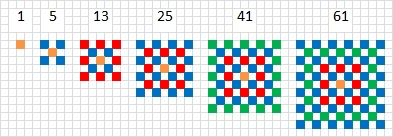545 is a centered square number because 16 and 17 are consecutive numbers and (16^2) + (17^2) = 545.

It is probably less exciting that (23^2) + (4^2) = 545.

545 is the hypotenuse of four Pythagorean triples. Which of these triples are primitives and which of them aren’t? The ones with greatest common factors greater than one are not primitive:

• 33-544-545
• 184-513-545
• 300-455-545
• 327-436-545Print the puzzles or type the solution on this excel file: 10 Factors 2015-07-06

—————————————————————————————————

• 545 is a composite number.
• Prime factorization: 545 = 5 x 109
• The exponents in the prime factorization are 1 and 1. Adding one to each and multiplying we get (1 + 1)(1 + 1) = 2 x 2 = 4. Therefore 545 has exactly 4 factors.
• Factors of 545: 1, 5, 109, 545
• Factor pairs: 545 = 1 x 545 or 5 x 109
• 545 has no square factors that allow its square root to be simplified. √545 ≈ 23.345235—————————————————————————————————